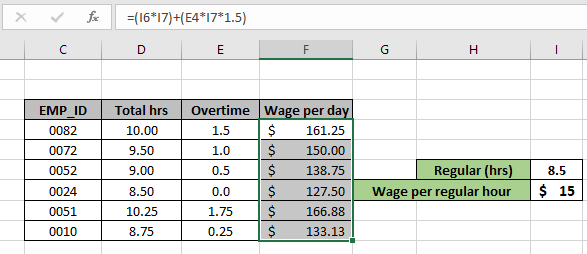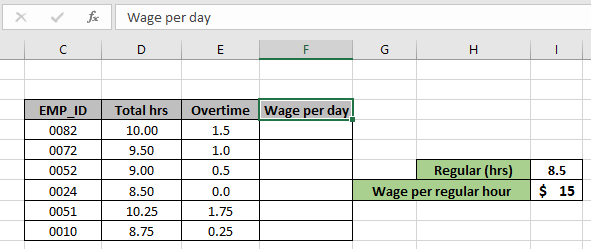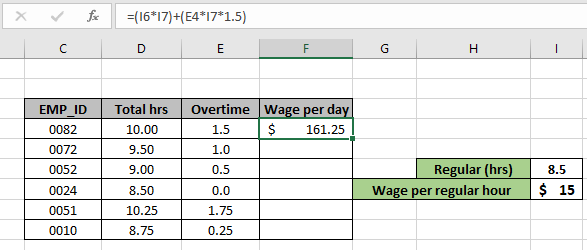# Calculate Overtime amount using excel formulaIn this article, we will learn how to calculate overtime wages using the excel formula.

Sometimes we need to calculate the wages of the day including the employees overtime. To find the total wage for a particular employee including its overtime we will use the overtime formula below.

Generic Formula:

= ( reg_hrs * wage/hr ) + ( over_time * wage/hr * compensation )

reg_hrs : regular hours

wage/hr : wage per hour

over_time : over time

compensation : extra wage to compensate for overtime

### Example: Calculating Wages With Overtime Wage in Excel

Here we have some data and compensation is 1.5 times the wage per hour.Use the formula in the G6 cell:

= ( I6 * I7 ) + ( E4 * I7 * 1.5 )

Explanation:

• ( I6 * I7 ) calculates the wage for the regular hour of the day.
• ( E4 * I7 * 1.5 ) calculates the compensation wage p[er customer.As you can see the wage for the whole day for the employee ID 0082.

Now copy the formula to the other cells to get the Wage per day for other Employees. Use Ctrl + D or drag down option to get results.Hope you understand how to Calculate Overtime wage using excel formula in Excel. Explore more articles in Excel lookup value here. Please feel free to state your queries below in the comment box. We will certainly help you. If you liked our blogs, share it with your friends on Facebook. And also you can follow us on Twitter and Facebook. We would love to hear from you, do let us know how we can improve, complement or innovate our work and make it better for you.

Related Articles

Use INDEX and MATCH to Lookup Value : INDEX & MATCH function to look up value as required.

SUM range with INDEX in Excel : Use INDEX function to find the SUM of the values as required.

How to use the INDEX function in Excel : Find the INDEX of array using the INDEX function explained with example.

How to use the MATCH function in Excel : Find the MATCH in the array using the INDEX value inside MATCH function explained with example.

How to use LOOKUP function in Excel : Find the lookup value in the array using the LOOKUP function explained with example.

How to use the VLOOKUP function in Excel : Find the lookup value in the array using the VLOOKUP function explained with example.

Popular Articles

50 Excel Shortcut to Increase Your Productivity

Edit a dropdown list

Absolute reference in Excel

If with wildcards

Vlookup by date

Terms and Conditions of use

The applications/code on this site are distributed as is and without warranties or liability. In no event shall the owner of the copyrights, or the authors of the applications/code be liable for any loss of profit, any problems or any damage resulting from the use or evaluation of the applications/code.